## Statistics Answers

#### Contact Us

You can e-mail us your problems for a free quote.

Sample Problem. The time it takes an international telephone operator to place an overseas phone call is normally distributed with mean 45 seconds and standard deviation 10 seconds.

a. What is the probability that my call will go through in less than 1 minute?

b. What is the probability that I will get through in less than 40 seconds?

c. What is the probability that I will have to wait more than 70 seconds for my call to go through?

Solution:

a. First thing is, let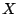be the time it takes to the telephone operator to place an overseas phone call. The probability we are looking for is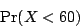In order to compute this probability, we need to normalizein the following way. We know that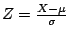has standard normal distribution, so we compute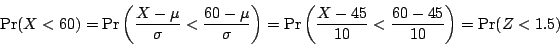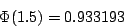(notice that since the mean is given in seconds, we have to write 1 minute as 60 seconds)

b. Same as before, letbe the time it takes to the telephone operator to place an overseas phone call. The probability we are looking for is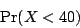In order to compute this probability, we need to normalizein the same way we did before. We know thathas standard normal distribution, so we compute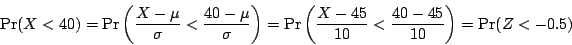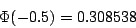c.is the time it takes to the telephone operator to place an overseas phone call. The probability we are looking for is equal to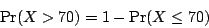As before, we need to normalize. We get that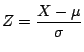has standard normal distribution, and we compute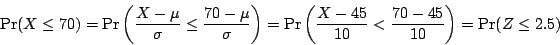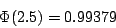and therefore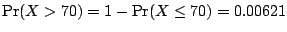.